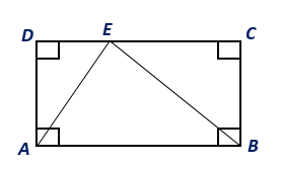Chapter 8.CT, Problem 4CT### Elementary Geometry for College St...

6th Edition
Daniel C. Alexander + 1 other
ISBN: 9781285195698

#### Solutions

Chapter
Section### Elementary Geometry for College St...

6th Edition
Daniel C. Alexander + 1 other
ISBN: 9781285195698
Textbook Problem
4 views

# If the area of rectangle ABCD is 46 cm2, find the area of ∆ ABE.                                   _To determine

To find:

The area of the triangle ABE.

Explanation

Given:

The area of rectangle ABCD is 46 cm2.

Postulate:

1. The area A of a rectangle whose base has length b and whose altitude has the length h is given by A=bh.

2. The area A of a triangle whose base has length b and whose altitude has the length h is given by A=12bh

### Still sussing out bartleby?

Check out a sample textbook solution.

See a sample solution

#### The Solution to Your Study Problems

Bartleby provides explanations to thousands of textbook problems written by our experts, many with advanced degrees!

Get Started

#### Convert the expressions in Exercises 6584 to power form. 35x5x8+72x3

Finite Mathematics and Applied Calculus (MindTap Course List)

#### In Exercises 63-68, rationalize the denominator. 63. 32x

Applied Calculus for the Managerial, Life, and Social Sciences: A Brief Approach

#### True or False: If a number p is in the interval of convergence of then so is the number .

Study Guide for Stewart's Single Variable Calculus: Early Transcendentals, 8th

#### True or False: div curl F = 0.

Study Guide for Stewart's Multivariable Calculus, 8th